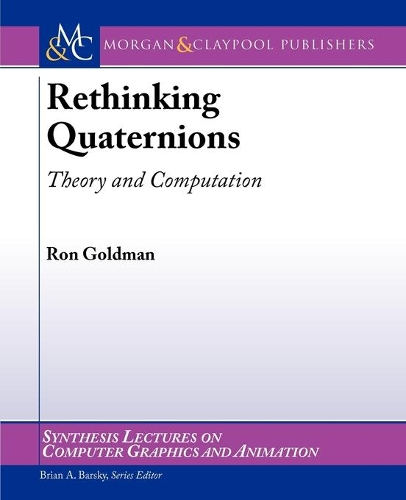•# Rethinking Quaternions - Synthesis Lectures on Computer Graphics and Animation (Paperback)

(author), (series editor)
£45.50
Paperback 157 Pages / Published: 30/10/2010
• We can order this

Usually dispatched within 3 weeks

Quaternion multiplication can be used to rotate vectors in three-dimensions. Therefore, in computer graphics, quaternions have three principal applications: to increase speed and reduce storage for calculations involving rotations, to avoid distortions arising from numerical inaccuracies caused by floating point computations with rotations, and to interpolate between two rotations for key frame animation. Yet while the formal algebra of quaternions is well-known in the graphics community, the derivations of the formulas for this algebra and the geometric principles underlying this algebra are not well understood. The goals of this monograph are to provide a fresh, geometric interpretation for quaternions, appropriate for contemporary computer graphics, based on mass-points; to present better ways to visualize quaternions, and the effect of quaternion multiplication on points and vectors in three dimensions using insights from the algebra and geometry of multiplication in the complex plane; to derive the formula for quaternion multiplication from first principles; to develop simple, intuitive proofs of the sandwiching formulas for rotation and reflection; to show how to apply sandwiching to compute perspective projections.

In addition to these theoretical issues, we also address some computational questions. We develop straightforward formulas for converting back and forth between quaternion and matrix representations for rotations, reflections, and perspective projections, and we discuss the relative advantages and disadvantages of the quaternion and matrix representations for these transformations. Moreover, we show how to avoid distortions due to floating point computations with rotations by using unit quaternions to represent rotations. We also derive the formula for spherical linear interpolation, and we explain how to apply this formula to interpolate between two rotations for key frame animation. Finally, we explain the role of quaternions in low-dimensional Clifford algebras, and we show how to apply the Clifford algebra for R3 to model rotations, reflections, and perspective projections. To help the reader understand the concepts and formulas presented here, we have incorporated many exercises in order to clarify and elaborate some of the key points in the text.

Publisher: Morgan & Claypool Publishers
ISBN: 9781608454204
Number of pages: 157
Weight: 314 g
Dimensions: 235 x 187 x 9 mm